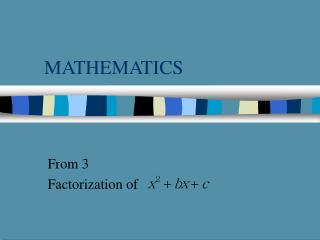DownloadDownload PresentationMATHEMATICS

# MATHEMATICS

Télécharger la présentation## MATHEMATICS

- - - - - - - - - - - - - - - - - - - - - - - - - - - E N D - - - - - - - - - - - - - - - - - - - - - - - - - - -
##### Presentation Transcript

1. MATHEMATICS From 3 Factorization of

2. Introduction • Objectives:Encouraged to practice thinking skills such as ‘trial and error’ or ‘use equations’. • Target:After the lesson, students should be able to do simple factorization. • Duration:30 mins.

3. Introduction • Target group:Form 3 students. • Assumption:Students are expected to know how to solve simple linear equations.

4. What shall we get when we expand ?

5. What shall we get when we expand ? + qx

6. What shall we get when we expand ? + px + qx

7. What shall we get when we expand ? + px + pq + qx

8. What shall we get when we expand ? Simplify by taking the x out. + px + pq + qx

9. What shall we get when we expand ? Answer : + pq + (p + q) x

10. Consider this example Expand : (x + 2)( x + 3)

11. Consider this example Expand : (x + 2)( x + 3) + 3x

12. Consider this example Expand : (x + 2)( x + 3) + 3x + 2x

13. Consider this example Expand : (x + 2)( x + 3) + 2x3 + 3x + 2x

14. Consider this example Expand : (x + 2)( x + 3) Again, simplify by taking the x out. + 2x3 + 3x + 2x

15. Consider this example Expand : (x + 2)( x + 3) Therefore the answer is + (2 + 3) x + 2x3

16. Compare with the previous example (x + 2)( x + 3) + (2 + 3) x + 2x3 = + (p + q) x + pq =

17. Now, what are the answers for the following expression ? (x+4)(x+7) = x2+ ( ? )x + ( ? ) The answer is EASY!! (x+4)(x+7) = x2+ ( )x + ( ) 11 28 4x7 4+7

18. To do it the other way round is harder. Can you do this? = (x + ? ) (x + ? ) The answer is = (x + ) (x + ) 2 5 2 x 5 2 + 5

19. Steps to factorize : = (x + ? ) (x + ? ) Fist draw two brackets

20. Steps to factorize : = (x + ? ) (x + ? ) Then consider the factors of 10 That is :1 x 10 or 2 x 5

21. Steps to factorize : = (x + ? ) (x + ? ) Try 1 and 10 = (x + ) (x + ) 1 10 WRONG 1+10 is not equal to 7 therefore this is...

22. Steps to factorize : = (x + ? ) (x + ? ) Try 2 and 5 = (x + ) (x + ) 2 5 2+5 is equal to 7 therefore this is... CORRECT

23. Interesting web-sites containing materials of factorization. • http://mathforum.com/mathworld/ • http://www.crosswinds.net/~despot/felecia/default_html/index.html • http://www.algebrahelp.com/

24. THE END